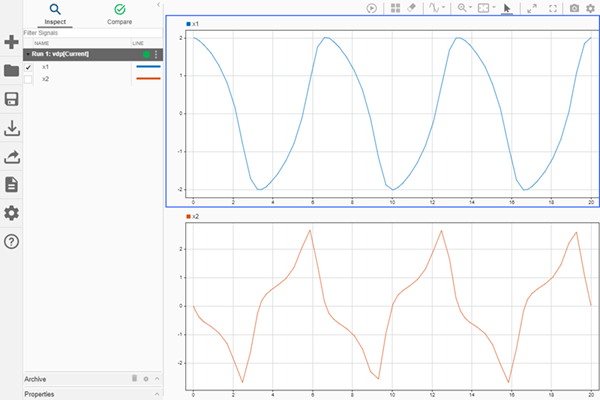# plotOnSubPlot

Plot `Simulink.sdi.Signal` object on Simulation Data Inspector subplot

## Syntax

``plotOnSubplot(sig,r,c,checked)``

## Description

example

``` `plotOnSubplot(sig,r,c,checked)` plots or clears the signal that corresponds to the `Simulink.sdi.Signal` object `sig` on the subplot specified by `r` and `c`.```

## Examples

collapse all

This example demonstrates how to access the `Simulink.sdi.Run` object for a Simulation Data Inspector run created by logging signals. From the `Simulink.sdi.Run` object you can get `Simulink.sdi.Signal` objects that contain the logged signal data and metadata. You can use the `Signal` objects and the `plotOnSubPlot` function to plot the data in the Simulation Data Inspector.

Create a Simulation Run and Access the `Run` Object

The `ex_vdp` model logs two signals. To create a simulation run containing the logged data, simulate the model.

`sim('ex_vdp');`

The Simulation Data Inspector keeps track of runs by assigning a unique numeric run ID to each run created by simulation, importing data, or opening a session. To access the run object for the simulation you just performed, use the `Simulink.sdi.getAllRunIDs` function and take the last run ID in the returned vector.

```runIDs = Simulink.sdi.getAllRunIDs; runID = runIDs(end);```

Once you have the run ID for the run, you can use the `Simulink.sdi.getRun` function to get the `Simulink.sdi.Run` object that corresponds to the run. You can use the `Run` object to check the metadata associated with the run, including the number of signals in the run.

```vdpRun = Simulink.sdi.getRun(runID); vdpRun.SignalCount```
```ans = int32 2 ```

Plot Data Using `Signal` Objects

Use the `getSignalByIndex` function to access signals from the `Run` object, `fuelRun`.

```signal1 = getSignalByIndex(vdpRun,1); signal2 = getSignalByIndex(vdpRun,2);```

Use the `Simulink.sdi.setSubPlotLayout` function to specify a 3-by-1 layout.

`Simulink.sdi.setSubPlotLayout(2,1)`

Before plotting the data, use the `Simulink.sdi.clearAllSubPlots` function to clear any data that is already plotted.

`Simulink.sdi.clearAllSubPlots`

Plot one signal on each subplot. To plot signals on the first subplot, you can set the `checked` property for the signal. To plot signals on subplots other than the first subplot, use the `plotOnSubPlot` function.

```signal1.Checked = true; plotOnSubPlot(signal2,2,1,true);```

View the Plotted Data

To view the plots you just created, open the Simulation Data Inspector using the `Simulink.sdi.view` function.## Input Arguments

collapse all

Signal to plot, specified as a `Simulink.sdi.Signal` object.

Row index for the subplot, specified as an integer between `1` and `8`, inclusive.

Example: `1`

Column index for the subplot, specified as an integer between `1` and `8`, inclusive.

Example: `2`

Plot or clear the signal on the subplot, specified as one of these values:

• `true` plots the signal on the subplot.

• `false` clears the signal from the subplot.

Data Types: `logical`

## Alternatives

You can use the Simulation Data Inspector UI to modify the plot layout and plotted signals. For more information, see Inspect Simulation Data.

## Version History

Introduced in R2017b